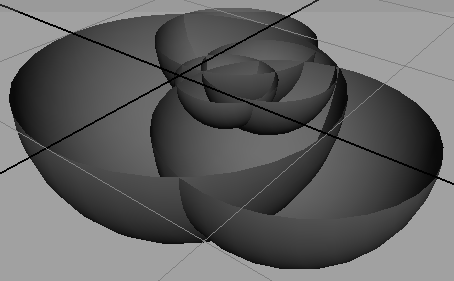### MelRecursion

#### Introduction

This tutorial addresses the issue of writing recursive procedures. A procedure is recursive when it calls or invokes itself. As a simple example consider the following procedure.

```    proc foo(){
sphere;
foo(); // call ourself
}```

The color coded sequence shown below indicates what happens when the procedure is executed. Each time `foo`() is executed it would call a "copy" of itself, which in turn would call another "copy" - and so on. Each recursion ie. copy of the procedure, occupies a small allocation of memory. Eventually Maya would use up its memory and terminate!

 ```proc foo(){ sphere; foo(); }``` ```proc foo(){ sphere; foo(); }``` ```proc foo(){ sphere; foo(); }```

#### Terminating Recursion

Recursive procedures must always be given a way of stopping the recursion process. Before they recursively call themself they test if a terminal condition is true or false. For example.

```    proc foo(int \$depth)
{
sphere;

// terminate the recursion...
if(\$depth == 0)
return;

foo(\$depth - 1); // call ourself
}```

In a mel script this new version of the `foo`() procedure might be used as follows,
`    foo(5);`

The variable `\$depth` determines the number of times recursion will occur. As soon as `\$depth` equals 0, recursion is terminated. The new terminating version of `foo`() is equivalent to using a for-loop:

```    for(int \$n = 0; \$n < 5; \$n++)
sphere;```

So why bother with recursion? Why not use a for-loop to repeat a particular action - such as creating a sphere?

#### Why Use Recursion?

Recursion is particulary helpful when modeling branching and/or nested structures that consist of self-similar shapes. For example, the space filling Hilbert curve shown in fig 1 was created with a recursive procedure.

##### Example 1 - knobby sphere

The cluster of spheres in figure 1 was created with the procedure shown in listing 1.Figure 1

Listing 1 (spheres.mel)

 ``` proc spheres(int \$depth, vector \$pnt, float \$rad) { if(\$depth == 0) return; // create a sphere sphere -p (\$pnt.x) 0 (\$pnt.z) -r \$rad -esw 180; // find a random location on the surface of the sphere \$pnt = unit(sphrand(1)) * \$rad; // recurse... knobby(\$depth - 1, \$pnt, \$rad * 0.75); }```

Line 7 includes the -esw flag (endSweep) flag so that hemi-spheres are produced. In addition the y coordinte is set to 0.0 to ensure the top edges of the spheres are aligned to the x-z plane. In this way it is easy to see that some "children" spheres are enclosed within the inner "parent" spheres.

For a more sophisticated examples of recursion refer to the tutorials "Mel: Recursion & Branching" and "Hilbert Curve: Mel Implementation"